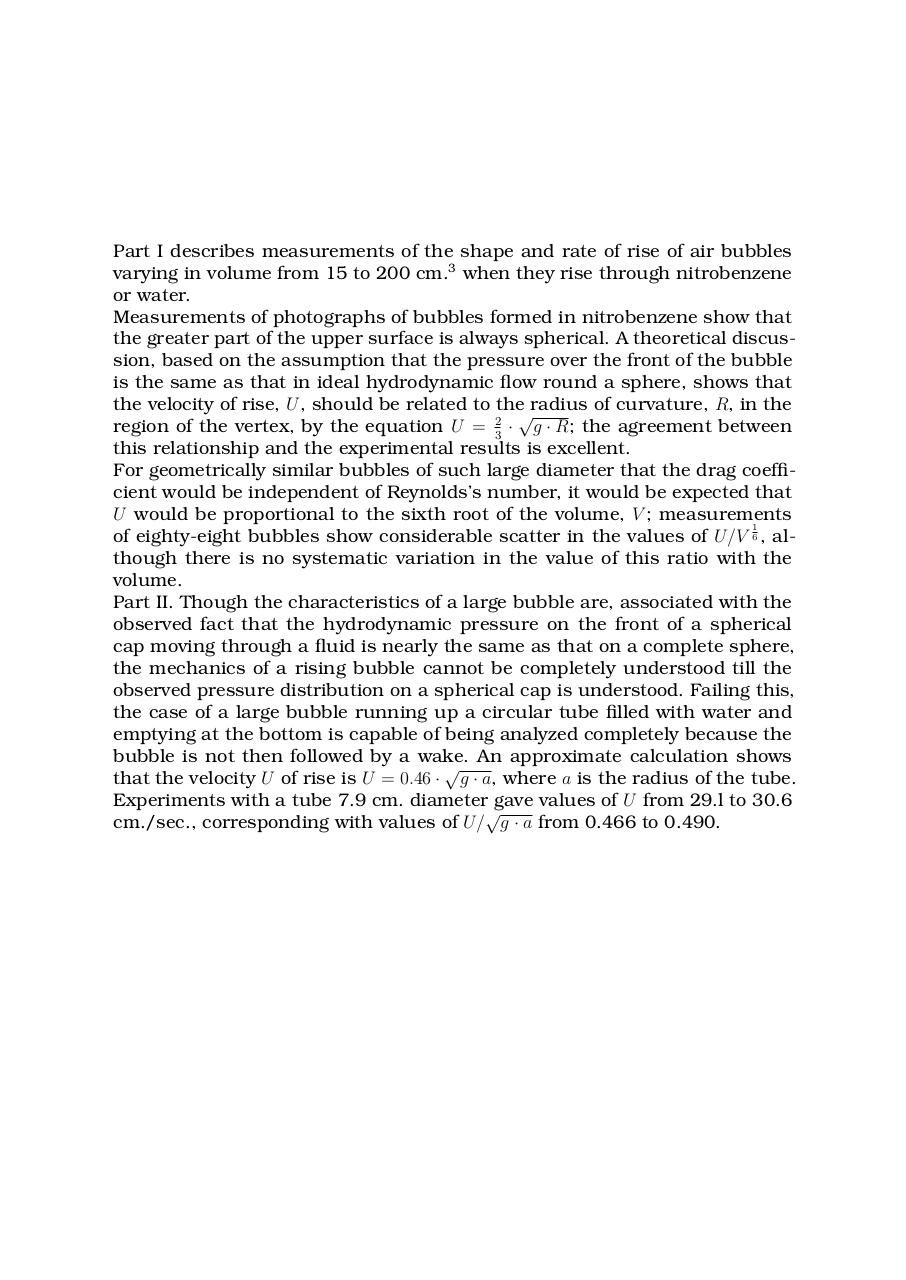# Taylor, G. I. The Mechanics of Large Bubbles Rising through Extended Liquids and through Liquids in Tubes.pdfPage 1 23425

#### Text preview

Part I describes measurements of the shape and rate of rise of air bubbles
varying in volume from 15 to 200 cm.3 when they rise through nitrobenzene
or water.
Measurements of photographs of bubbles formed in nitrobenzene show that
the greater part of the upper surface is always spherical. A theoretical discussion, based on the assumption that the pressure over the front of the bubble
is the same as that in ideal hydrodynamic flow round a sphere, shows that
the velocity of rise, U , should be related to the√radius of curvature, R, in the
region of the vertex, by the equation U = 23 · g · R; the agreement between
this relationship and the experimental results is excellent.
For geometrically similar bubbles of such large diameter that the drag coefficient would be independent of Reynolds’s number, it would be expected that
U would be proportional to the sixth root of the volume, V ; measurements
1
of eighty-eight bubbles show considerable scatter in the values of U/V 6 , although there is no systematic variation in the value of this ratio with the
volume.
Part II. Though the characteristics of a large bubble are, associated with the
observed fact that the hydrodynamic pressure on the front of a spherical
cap moving through a fluid is nearly the same as that on a complete sphere,
the mechanics of a rising bubble cannot be completely understood till the
observed pressure distribution on a spherical cap is understood. Failing this,
the case of a large bubble running up a circular tube filled with water and
emptying at the bottom is capable of being analyzed completely because the
bubble is not then followed by a wake. An approximate calculation shows

that the velocity U of rise is U = 0.46 · g · a, where a is the radius of the tube.
Experiments with a tube 7.9 cm. diameter gave values of U from 29.l to 30.6

cm./sec., corresponding with values of U/ g · a from 0.466 to 0.490.